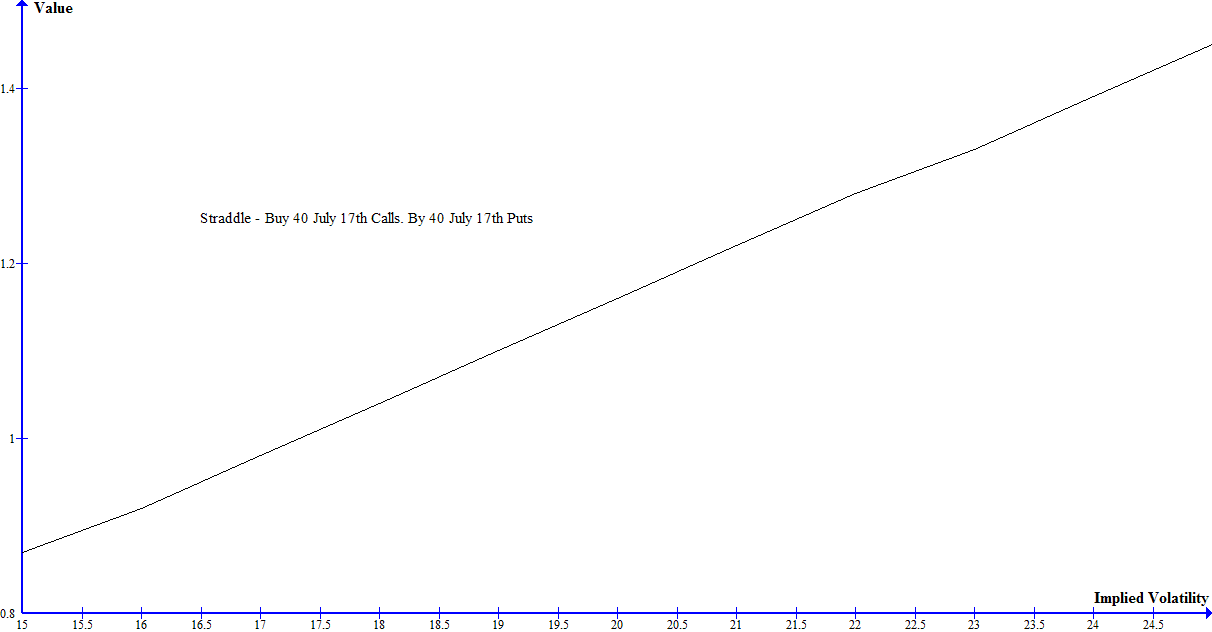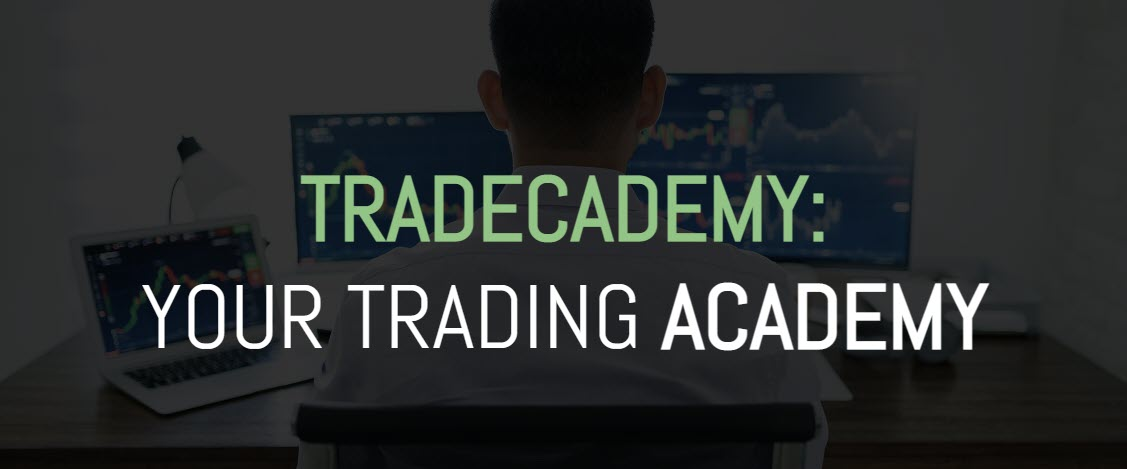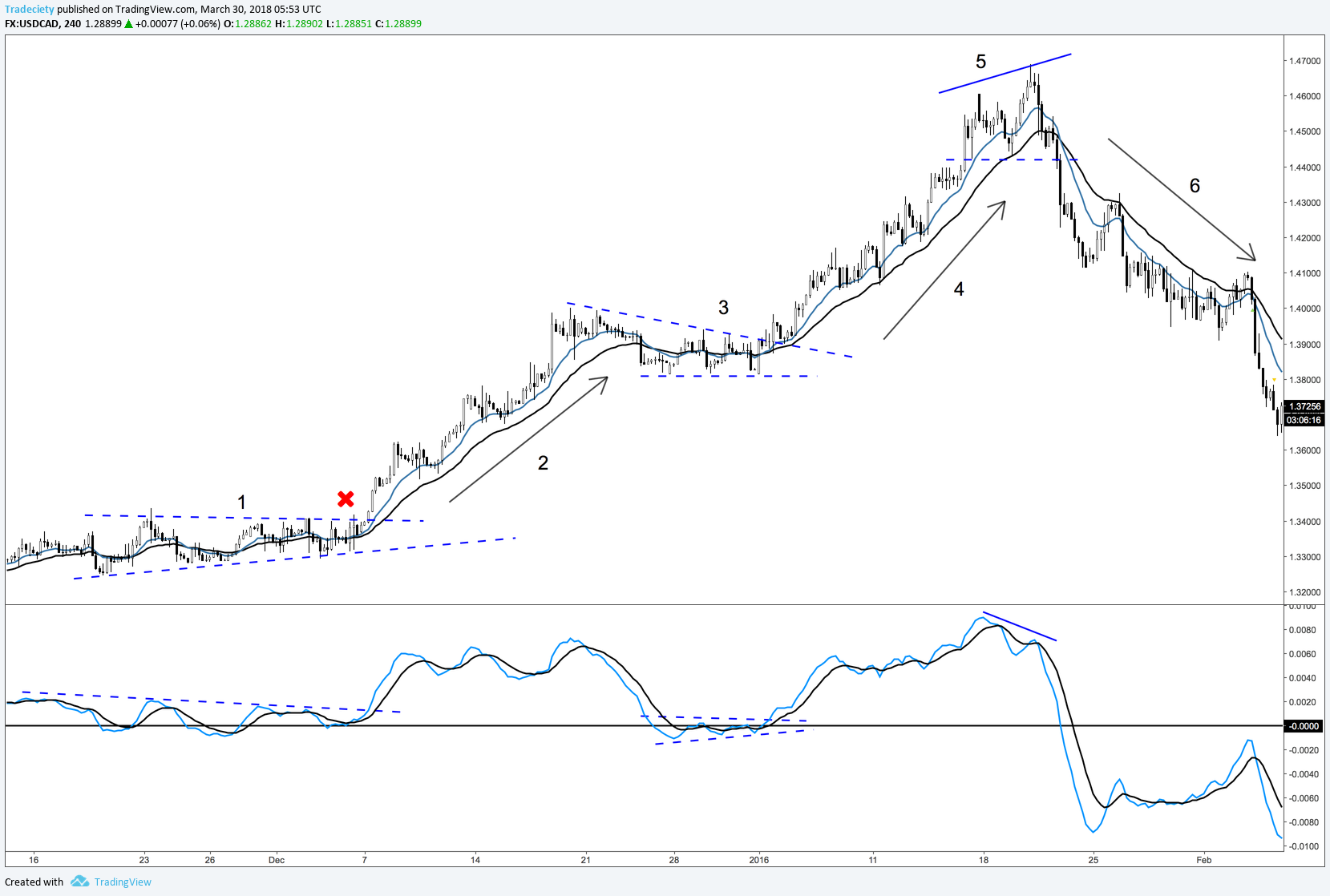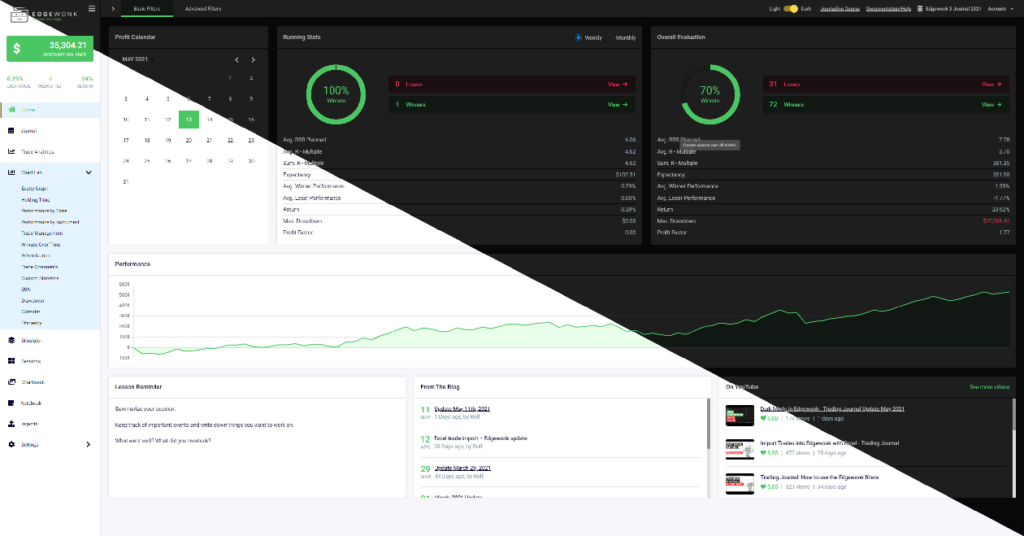The following article is a guest post from Larry W. from Options Strategies

There is a lot to learn when moving from trading stocks to trading stocks and options. With options you have more flexibility and opportunities to speculate. Options give a trader the ability to take advantage of more than just market direction.

In this article I am going to show how you can profit from an increase in the expected volatility of an underlying stock using options. I am also going to illustrate how you can manage your risk and reward, using three different types of option spreads.

### Implied volatility – The unknown factor of options trading

The first thing to understand when trading volatility with options is what implied volatility is. Implied volatility is the volatility that is backed out of an options price. This can be done, because it is the only unknown that is plugged into an option pricing model formula. The other factors that make up an option price are the relationship between the strike and the underlying price (intrinsic value), time to expiration, interest rates and dividends on some types of options. Think of it like solving an algebraic equation. You have the price on the right and a formula to get that price on the left, with one unknown. If you solve for the unknown, you get implied volatility. The implied volatility illustrates what the expected future volatility of the underlying is.

### Vega – Changes in implied volatility and option price changes

The next thing to know is how a change in implied volatility affects the price of an option. The measure for this is called vega. Vega is one of the “Greeks”. There are more Greeks, such as delta and theta, that measure how option prices move based on other factors. For this article we will concentrate on vega. Vega tells you how much an option contract price will move for each 1% move in implied volatility. Here is a graph that shows how Vega changes as the price of an underlying stock changes.

The closer to the money options have higher vegas than the more out of the money ones. Also, vega is positive for both puts and calls. So, if you buy an option you benefit from an increase in implied volatility. Conversely, if you sell an option, you benefit if it goes down.

In order to get the maximum punch from an increase in implied volatility, you would need to buy at the money puts and calls. This is called a long straddle. More specifically, you would need to buy an equal amount of at the money puts and calls, with the same expiration.

### Long Straddle – Get the most from a change in implied volatility

If Coca Cola stock is trading at 40 and you buy 5 July 17th 40 calls and 5 July 17th 40 puts on Coca Cola, you have executed a long straddle. At 15% implied volatility and 11 days to expiration the calls will be trading at .44 and the puts at .43. The trade will cost you \$870. Keeping all other factors the same (time to expiration, stock price and interest rates), if implied volatility climbs to 25% the calls will climb to .73 and the puts to .72. The position will be worth \$1,450. For a profit of \$580 attributable to a climb in implied volatility. Here is chart of implied vs. the combined position price.You are maximizing the sensitivity to a change in implied volatility by buying at the money options.

What if you do not want to risk as much on the trade and don’t want to have as much sensitivity to a change in implied volatility. You could purchase an equal amount of out of the money puts and calls with the same expiration. This is called a long strangle.

### Long Strangle – Risk less in exchange for less upside

If Coca Cola is trading at 40 and you buy 5 July 17th 41 calls and 5 July 17th 39 puts, you have executed a long strangle. At 15% implied volatility and 11 days to expiration the calls will be trading at .11 and the puts at .10. Out of the money options are going to be cheaper than at the money ones. The trade will cost you \$210. Keeping all other factors the same (time to expiration, stock price and interest rates), if implied volatility climbs to 25% the calls will climb to .34 and the puts to .32. The position will be worth \$660. For a profit of \$450 attributable to a climb in implied volatility. The change in price will be slightly less sensitive to a change in implied volatility. Here is a chart of implied vs. the combined position price.

The payout is less, so you risk less, but the implied volatility needs to increase more to net more of a profit. Remember, vega is lower for options that are out of the money.

If you want to have some exposure to a rise in implied volatility but want to limit your risk even more, you could execute a long strangle and simultaneously execute a short strangle that is more out of the money than the long strangle. So, you would buy out of the money calls and puts and sell even more out of the money calls and puts with the same expiration date and the same amount of contracts. This is called a reverse iron condor .

### Reverse Iron Condor – A more conservative strategy

The maximum loss will be the amount paid for the long strangle minus the premium received from the short strangle. This will be a net credit, since the closer to the money strangle will be more expensive. However, the spread will not cost as much as the straddle or strangle. Since the long strangle will have a higher vega than the short strangle, the position will benefit from a rise in implied volatility, but not as much as the straddle or strangle. It will also not lose as much from a drop in implied volatility.

Since the iron condor involves buying and selling so many positions, many traders will do what is called legging into the spread and buy the options over a period of time. For example, let’s say you have done your research and determined that the current implied volatility of the out of the money SPDR Euro Stoxx 50 ETF options are lower than they should be. There is an event you see that is not being captured in the market. Basically, you think the options are mispriced. The ETF is trading at 36. You buy 5 August 21 35 puts at .59 and August 21 37 calls at .62. With 46 days until expiration, the implied volatility is 20%. You get your event right after you enter the position. Implied volatility goes to 30%. If the underlying stays at the same price, the puts will be worth 1.07 and the call options will be worth 1.12. If you are assessment is that the options implied volatility could rise even more, but you want to adjust your risk, so as not to leave all that profit on the table, you may consider shorting a strangle more out of the money, fully implementing a reverse iron condor. Here is a chart of the strangle vs. the reverse iron condor. I am using 39 calls and 33 puts for the out of the money options to be sold.

You can see how the volatility risk reward profile of the trade is a lot different for the reverse iron condor vs. the long strangle. There are many reasons you may want to implement either one. You will need to decide when to implement any of these strategies based on your own trading style and appetite for risk.

In this article I isolated implied volatility as a trade. Here are some things to consider, that I did not talk about above, for sake of being able to explain trading volatility better.

• Implied volatility is not the same for all options, strikes, puts, calls and expirations. More out of the money options tend to have higher implied volatility than at the money ones, but that is not a hard rule.
• Consider liquidity when deciding which of these spreads to implement. At the money options tend to be more liquid than out of the money ones. Therefore, a long straddle will be easier to implement than a strangle. A reverse iron condor may be difficult to implement, especially for a thinly traded mid cap stock. Think of legging into your trades like I discussed above.
• We isolated changes in implied volatility. Other factors do affect the price of an option. Most importantly, the underlying price movement and time until expiration. These are measured by the “Greeks”, delta, gamma and theta. Each of which will be an article of their own.I set out with the goal to create the trading program that...